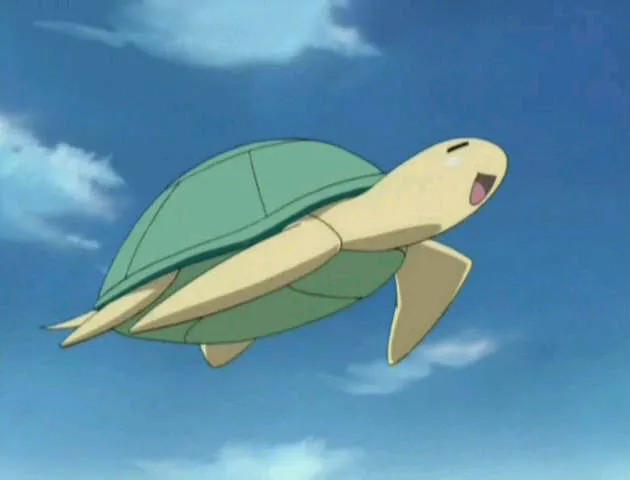# Lane of Things: Dance Room Activity

We used three types of sensors in order to catalog the usage of the dance room at our school and the impact the students have on the room.

IntermediateWork in progress20 hours250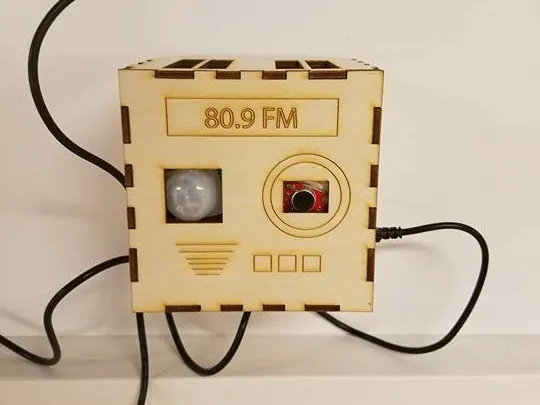## Things used in this project

### Hardware components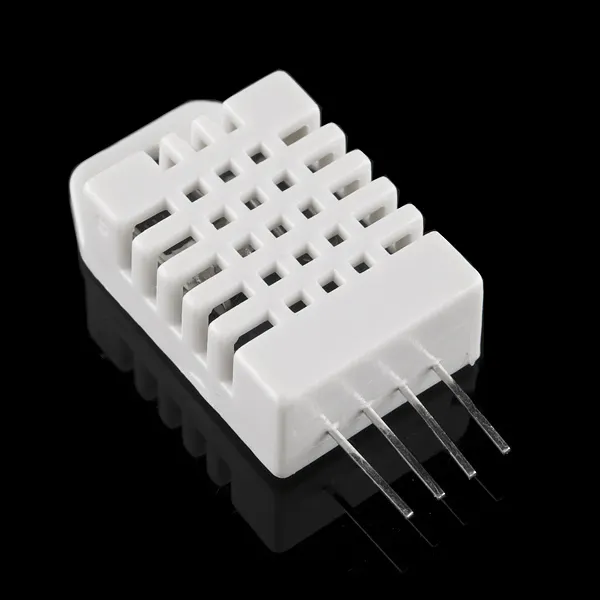DHT22 Temperature Sensor
×1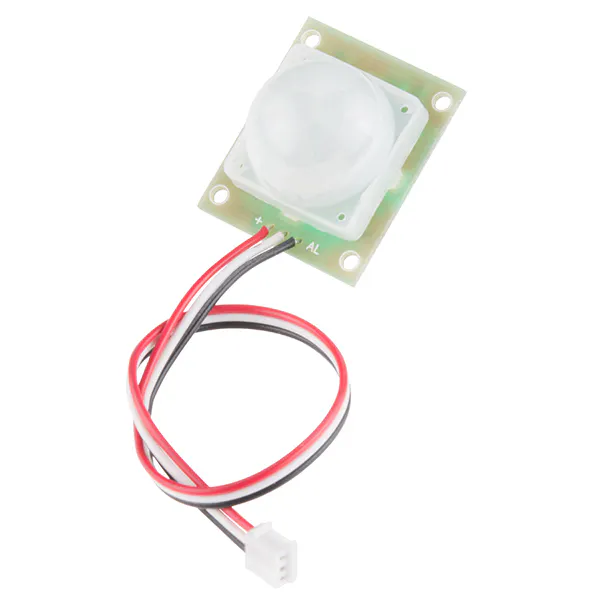PIR Motion Sensor (generic)
×1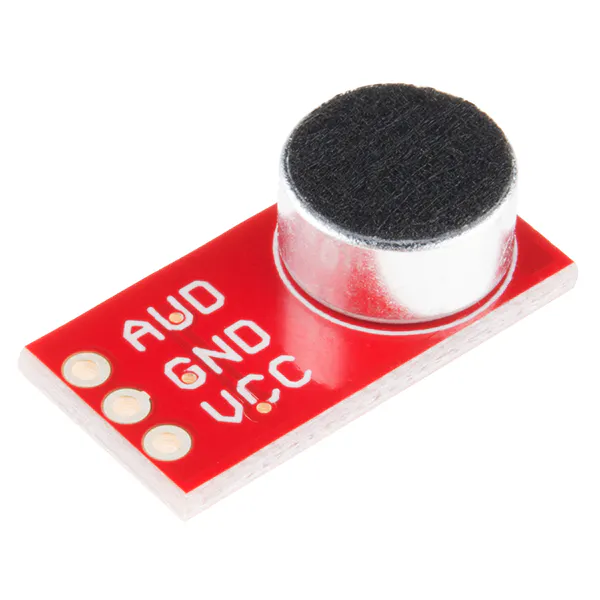SparkFun Electret Microphone Breakout
×1×1Particle Photon
×1

### Software apps and online services### Hand tools and fabrication machines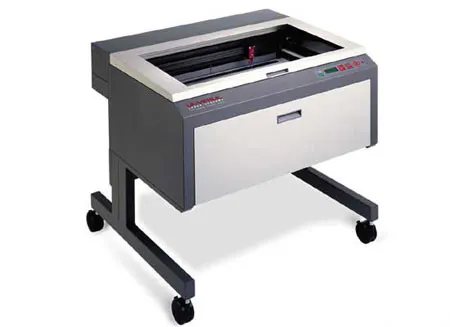Laser cutter (generic)Soldering iron (generic)

## Custom parts and enclosures

### Enclosure Design

We decided to make the enclosure to look like a radio since the location of the sensors/enclosure would be the dance room which makes the radio design completely relevant.

### Prototype Photos

This is a group of photos that show the prototypes and designing process of the enclosure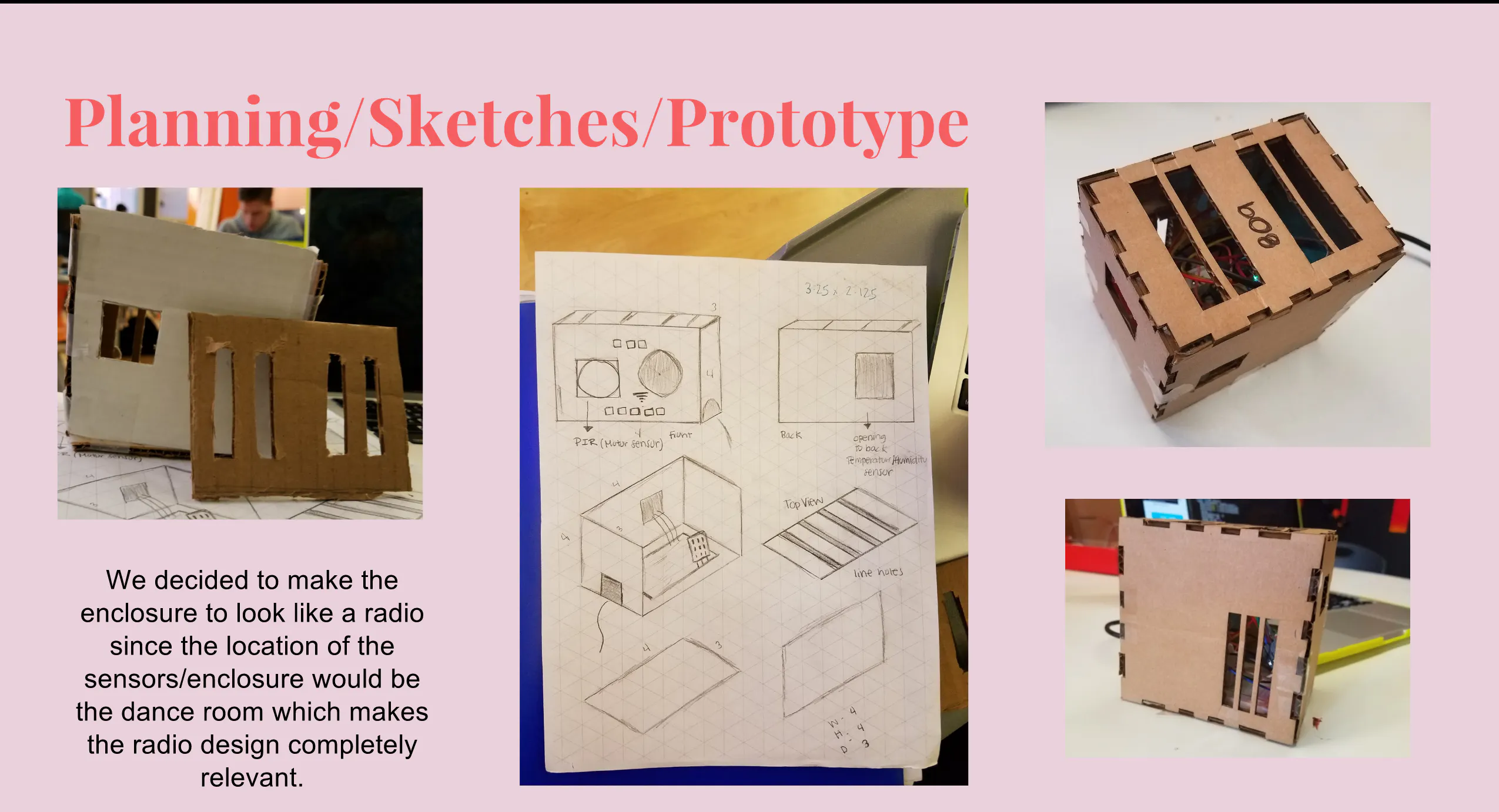### DATA CHART(Temperature)

A chart for temperature over time.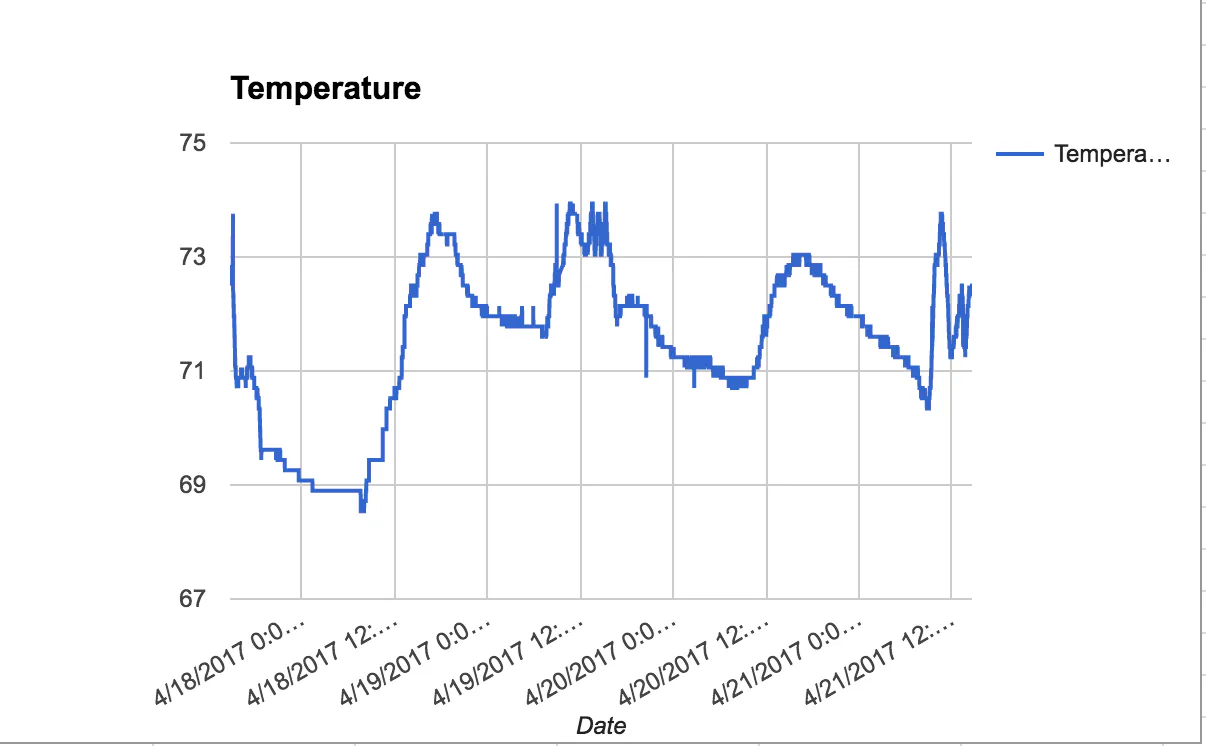### DATA CHART(Humidity)

A chart for temperature over time.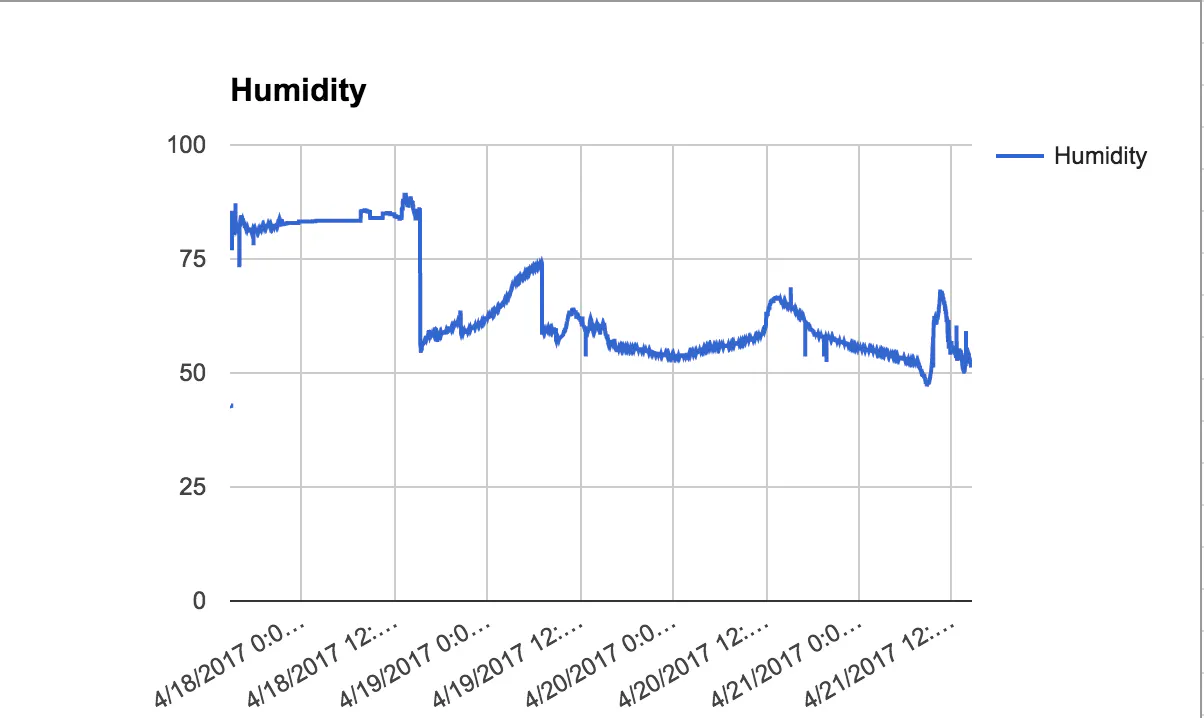### DATA CHART(Sound Average)

A chart showing the average of sound levels over time.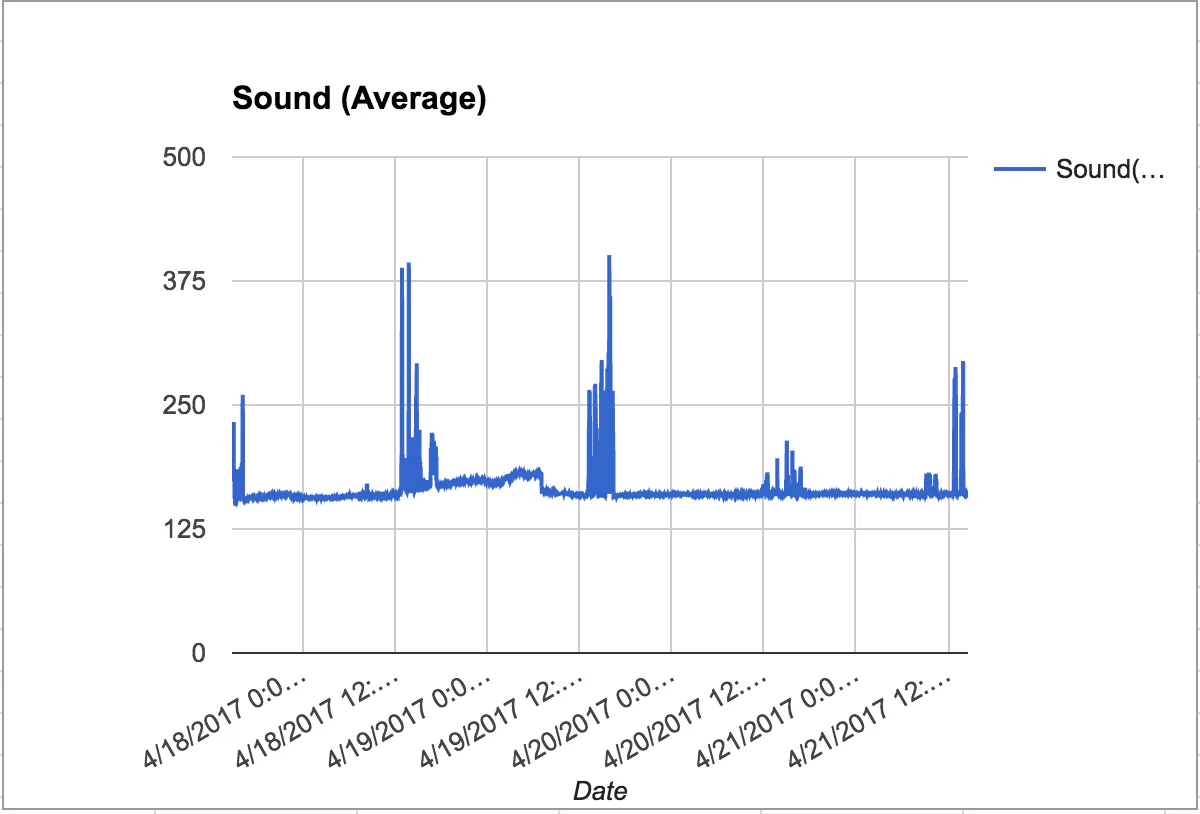### DATA CHART(Motion)

A chart showing whether motion was present (value = 1) over showing no motion (value = 0)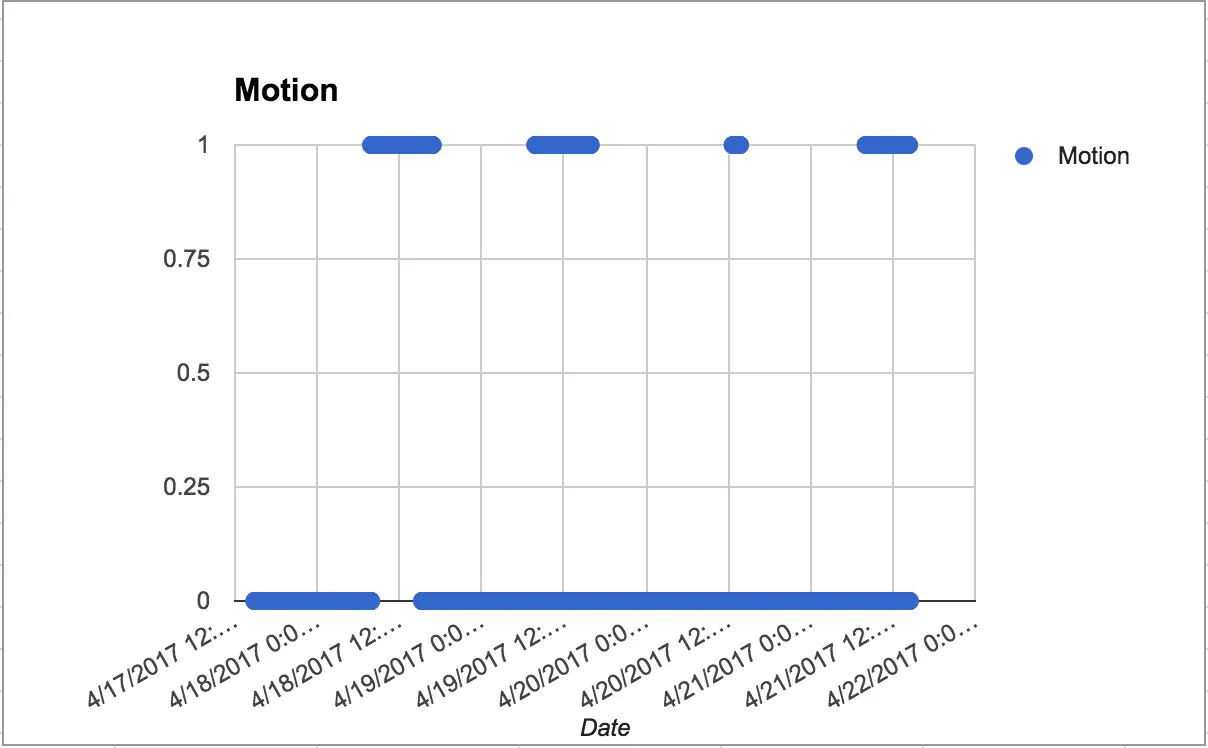### Data - Cleaned and Cut

Our set of data that shows the classroom, time, motion, sound, temperature and humidity.

### Picture of Enclosure Design

Picture of Enclosure Design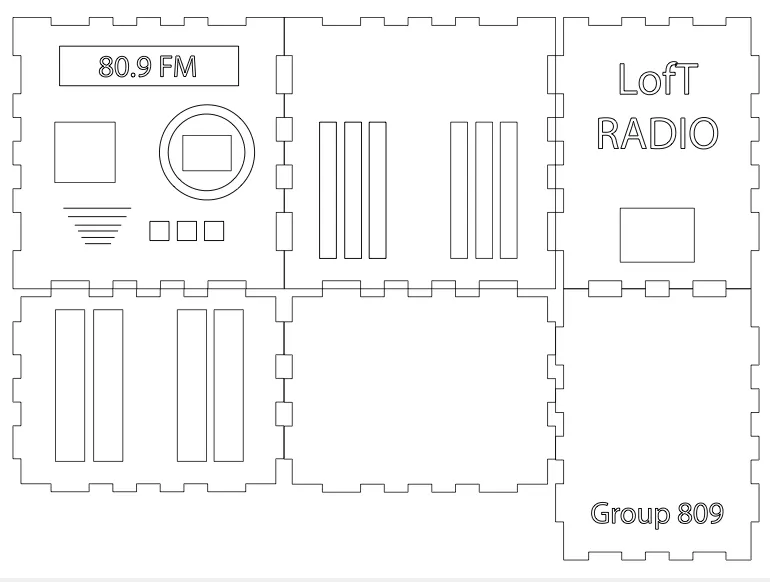## Schematics

### Project Design 2

This is the general Idea of how we hooked it up. We used different sensors with the same amount of connectors as substitutes for the PIR and sound detectors.

### Hookup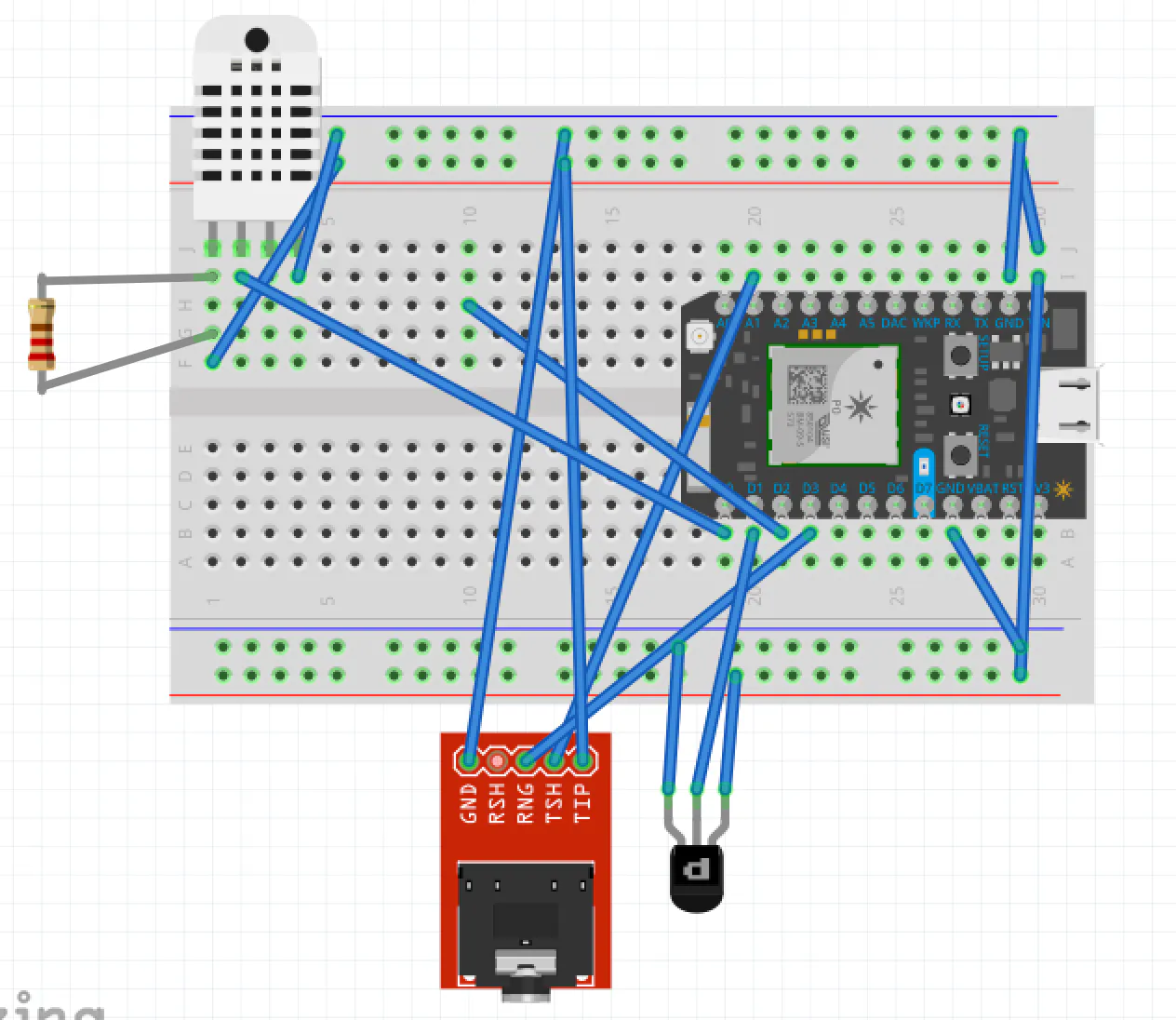## Code

### LofT-Com.ino

C/C++
```#include <Adafruit_DHT.h>

#define DHTPIN 4            // what pin we're connected to
#define DHTTYPE DHT22       // DHT 22 (AM2302)

DHT dht(DHTPIN, DHTTYPE);

double hum;                 // current hum
double temp;                // current temp
const long sample_rate = 50; // time between samples (in miliseconds)
const int array_size = 1200; // 1000/50=20 * 60=1200
int snd_array[array_size] = {};
int snd_max, prev_max = 0;
int snd_min, prev_min = 4096;
double snd_avg = 2048;
int inputPin = D2;               // choose the input pin (for PIR sensor)
int pirState = LOW;             // we start, assuming no motion detected
int val = 0;                    // variable for reading the pin status
int motVal = 0;

// Shift all the values right by 1
for(int i = array_size-1; i >= 1; i--)
{
snd_array[i] = snd_array[i-1];
if((snd_array[i] < snd_min) && (snd_array[i] != 0))
{
snd_min = snd_array[i];

}
if(snd_array[i] > snd_max)
{
snd_max = snd_array[i];

}
}

snd_array = value;

// Average array
float avg_sum = 0;
int size = 0 ;
for (int a=0; a <= array_size; a++)
{
if(snd_array[a] > 0)
{
size++ ;
avg_sum  = avg_sum + snd_array[a];
}
}
snd_avg = avg_sum / size;
}

digitalWrite(D7, HIGH);
} else {
digitalWrite(D7, LOW);
}
}

unsigned long now = millis();
Serial.print("Avg: "); Serial.println(snd_avg);
Serial.print("Min: "); Serial.println(snd_min);
Serial.print("Max: "); Serial.println(snd_max);
snd_avg = 0;
snd_min = 4096;
snd_max = 0;
snd_array[array_size] = {};
}
}

void setup() {
pinMode(inputPin, INPUT);     // declare sensor as input
Serial.begin(9600);
pinMode(DHTPIN, INPUT);
pinMode(A0, INPUT); // mic AUD connected to Analog pin 0
pinMode(D7, OUTPUT); // flash on-board LED

Particle.variable("hum", hum);
Particle.variable("temp", temp);
Particle.variable("snd_avg", snd_avg);
Particle.variable("snd_max", snd_max);
Particle.variable("snd_min", snd_min);
Particle.variable("motion", motVal);

}

void loop() {

delay(sample_rate);
double checkHum = dht.getHumidity();
double checkTemp = dht.getTempFarenheit();

if (checkHum > 0 && checkHum < 100)
hum = checkHum;
if (val == HIGH) {            // check if the input is HIGH
if (pirState == LOW) {
// we have just turned on
Serial.println("Motion detected!");
motVal = 1;
// We only want to print on the output change, not state
pirState = HIGH;
}
} else {
// digitalWrite(ledPin, LOW); // turn LED OFF
if (pirState == HIGH){
// we have just turned of
Serial.println("Motion ended!");
motVal = 0;
// We only want to print on the output change, not state
pirState = LOW;
}
}
if (checkTemp > 32 && checkTemp < 100)
temp = checkTemp;
if (val == HIGH) {            // check if the input is HIGH
if (pirState == LOW) {
// we have just turned on
Serial.println("Motion detected!");
motVal = 1;
// We only want to print on the output change, not state
pirState = HIGH;
}
} else {
// digitalWrite(ledPin, LOW); // turn LED OFF
if (pirState == HIGH){
// we have just turned of
Serial.println("Motion ended!");
motVal = 0;
// We only want to print on the output change, not state
pirState = LOW;
}
}

Serial.println("Temp: " + String(checkTemp));
Serial.println("Hum: " + String(checkHum));
Serial.println("Mot: "+String(motVal));
}
```

## Credits

### Gaudwin Timoteo

1 project • 0 followers